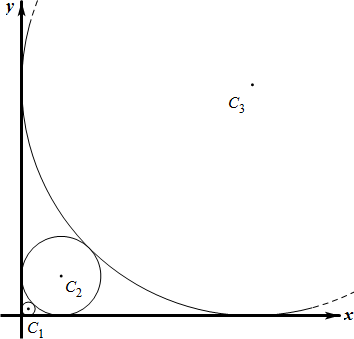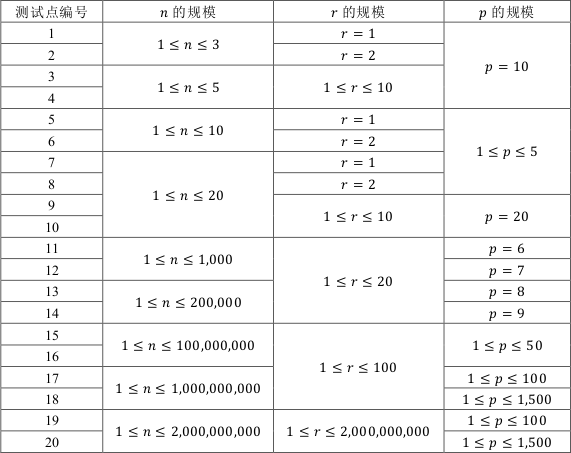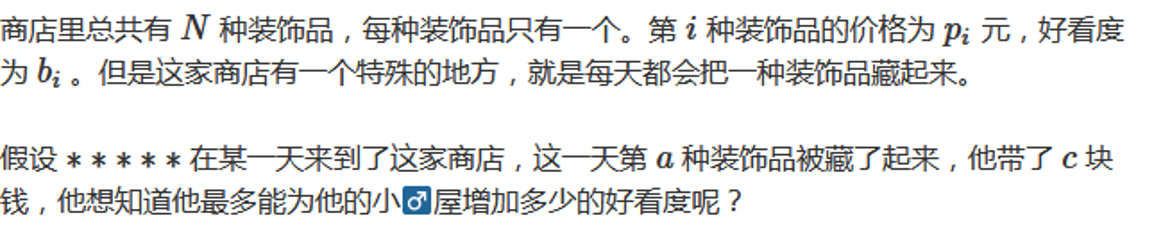# YZOJ P4621 [CSP-S 2019 四校联训 Round 1]生日悖论

• ### 数据规模与约定

$$1 \leq N \leq 10^{18}$$，$$2 \leq k \leq 10^{18}$$ 。

# YZOJ P3033 背包

• ### 题目描述

（即每种物品按照 $$1,2,3, \cdots ,b_i$$ 编号的方案数和不编号的方案数。）

# YZOJ P2443 回文子序列

• ### 样例说明

“”（空串）、”a”、”aa”、”aka”、”f”、”ff”、”k”、”kak”、”kk”、”t”

…

# YZOJ P1868 土地划分

• ### 题目描述

1，对于城市 $$i$$ ，如果它划分给 $$A$$ 国，将得到 $$VA[i]$$ 的得分；划分给 $$B$$ 国，将得到 $$VB[i]$$ 的得分。

2，对于一条公路 $$i$$ ，如果它连接两个 $$A$$ 国的城市，将得到 $$EA[i]$$ 的得分；连接两个 $$B$$ 国的城市，将得到 $$EB[i]$$ 的得分；否则，这条公路将失去意义，将扣除 $$EC[i]$$ 的得分。

• ### 数据规模与约定

$$100\%$$ 的数据 $$N \leq 10000, M \leq 40000$$；

…

…

# YZOJ P2697 画圆

• ### 题目描述

$$Alkri$$ 想在平面直角坐标系的第一象限中依次画 $$n$$ 个与两坐标轴均相切的圆，其中，第 $$1$$ 个圆的半径为 $$r$$，之后的每个圆都比上一个圆大，且与上一个圆相切，也就是说，对所有整数 $$2 \leq i \leq n$$，第 $$i$$ 个圆的半径大于第 $$i-1$$ 个圆的半径且与第 $$i-1$$个圆相切。• ### 数据规模与约定…

# [2017-2018 Petrozavodsk WC] J. Subsequence Sum Queries

• ### 题目描述…